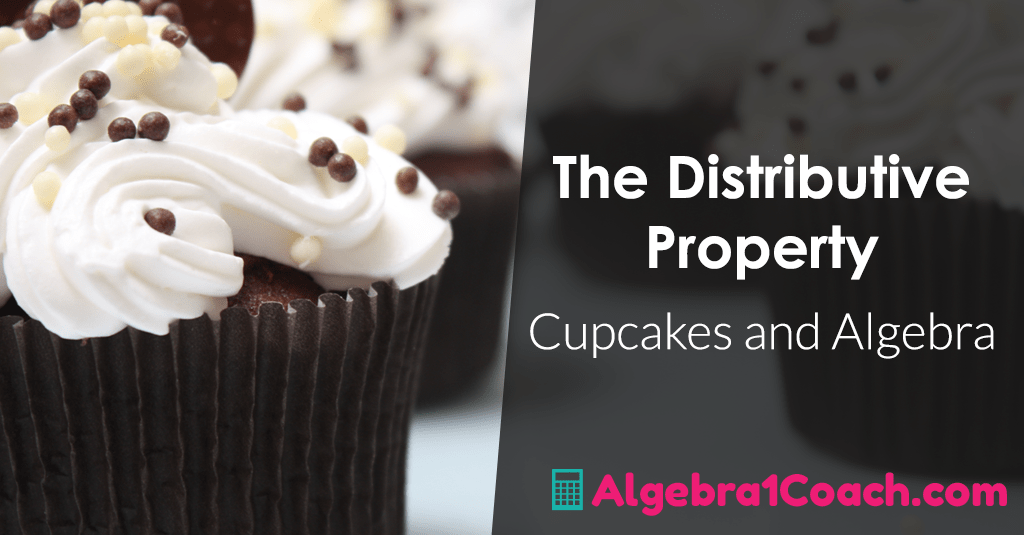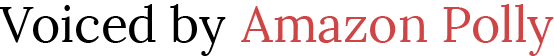# The Distributive Property Activity – Cupcakes and Algebra

 Would You Rather Listen to the Lesson?Okay, this lesson doesn't really require cupcakes but they can help! Before you ever teach the students the mathematical definition and how to use the Distributive Property you need to make sure they have an everyday definition and understanding of the word Distribute.

## Distributive Property Activities - Pass Them Out!

What does it mean to distribute something. I always use cupcakes as an example (and yes, I have brought them in to demonstrate). I ask the class would it be fair if I only passed out my cupcakes to the first row of students?

Of course they emphatically say no (unless they were in the first row). I ask them, why not?

They tell me because to be fair I need to pass them out to everyone. I agree with them and then go into the mathematical use of the Distributive property.

If I only multiply or distribute the x to the "first term" (referring back to the first row of students) in x(a+b). I would get ax + b which wouldn't be fair to the "second term" or in this case b. So, to be fair we would have to Distribute the x to both the a and the b to get ax+bx.

## Teaching the Distributive Property - Was Fun!

There you have it! They will never make the most common mistake with the Distributive Property ever again! Then when I teach them to distribute multiple terms to multiple terms like when multiplying two binomial terms together. I refer back to the cup cakes and ask if I brought in Cupcakes and Milk would it be fair to only pass one out to the first row and one to the second or both to the first row and not the second.

The easiest way to get them to understand the Distributive Property is to get them to understand it has to be fair across the board for all things being distributed and all thing receiving the distribution.

# Here are your Free Distributive Property Activities for this Lesson!

## The Distributive Property Worksheets PDF

1.) 1-7 Assignment - The Distributive Property (FREEBIE)

2.) 1-7 Bell Work - The Distributive Property (FREEBIE)

3.) 1-7 Guide Notes SE - The Distributive Property (FREEBIE)

4.) 1-7 Guide Notes TE - The Distributive Property (FREEBIE)

5.) 1-7 Lesson Plan - The Distributive Property (FREEBIE)

7.) 1-7 Slide Show - The Distributive Property (FREEBIE)

## The Distributive Property Worksheets - Word Docs & PowerPoints

To gain access to our editable content Join the Algebra 1 Teacher Community!
Here you will find hundreds of lessons, a community of teachers for support, and materials that are always up to date with the latest standards.# Guided Notes the Distributive Property

DISTRIBUTIVE PROPERTY

For any numbers 𝒂, 𝒃, and 𝒄, the product of 𝒂 and (𝒃 + 𝒄) is:

### (𝒃 + 𝒄)𝒂=𝒃𝒂+𝒄𝒂

For any numbers 𝒂, 𝒃, and 𝒄, the product of 𝒂 and (𝒃 − 𝒄) is:

## Sample Problem 1:

Rewrite using the distributive property, then evaluate.

𝟑(𝟏𝟐 + 𝟏)

= 𝟑 ⋅ 𝟏𝟐 + 𝟑 ⋅ 𝟏

= 𝟑𝟔 + 𝟑

= 𝟑𝟗

(𝟓 + 𝟖)𝟏𝟎

= 𝟓 ⋅ 𝟏𝟎 + 𝟖 ⋅ 𝟏𝟎

= 𝟓𝟎 + 𝟖𝟎

= 𝟏𝟑𝟎

𝟐(𝟏𝟐 − 𝟐𝟐)

= 𝟐 ⋅ 𝟏𝟐 − 𝟐 ⋅ 𝟐𝟐

= 𝟐𝟒 − 𝟒𝟒

= −𝟐𝟎

(𝟏𝟐 + 𝟑)𝟔

= 𝟏𝟐 ⋅ 𝟔 + 𝟑 ⋅ 𝟔

= 𝟕𝟐 + 𝟏𝟖

= 𝟗𝟎

## What Are Like Terms?

A TERM is a number, a variable or a product or quotient of numbers and variables.

LIKE TERMS are terms that contain the same variables, with corresponding variables having the same power.

## Simplifying Expressions:

The Distributive property is used to combine like terms by adding their coefficients. A simplified expression must not have grouping symbols and fractions are reduced to its lowest term.

## Sample Problem 2:

Simplify.

a.) 𝟏𝟐𝒙 + 𝟒𝒙
= 𝟏𝟔𝒙
b.) 𝟑𝒙² + 𝟒 − 𝒙²
= 𝟐𝒙² + 𝟒

c.) 𝟏 − 𝟐(𝟐 + 𝟑𝒙)
= 𝟏 − 𝟐(𝟐) − 𝟐(𝟑𝒙)
= 𝟏 − 𝟒 − 𝟔𝒙 = −𝟑 − 𝟔𝒙

d.) −𝟐(𝒙² + 𝟑𝒙 − 𝟐) + 𝟐𝒙
= −𝟐(𝒙²) − 𝟐(𝟑𝒙) − 𝟐(−𝟐) + 𝟐𝒙
= −𝟐𝒙² − 𝟔𝒙 + 𝟒 + 𝟐𝒙
= −𝟐𝒙² − 𝟒𝒙 + 𝟒

e.) 𝟐(𝒙 − 𝟒𝒚) + 𝟑(𝟐𝒙 + 𝟒𝒚)
= 𝟐(𝒙) − 𝟐(𝟒𝒚) + 𝟑(𝟐𝒙) + 𝟑(𝟒𝒚)
= 𝟐𝒙 − 𝟏𝟐𝒚 + 𝟔𝒙 + 𝟏𝟐𝒚 = 𝟖𝒙

## Sample Problem 3:

Manny runs a restaurant. One day, a total of 60 steaks are sold. Each steak costs \$16.95 and Manny received an average tip of \$1 for each steak he sold. Write the expression that determines the total amount he earned. How much did Manny earn?

𝟔𝟎(𝟏𝟔. 𝟗𝟓 + 𝟏)

= 𝟔𝟎(𝟏𝟕. 𝟗𝟓)

= \$𝟏,𝟎𝟕𝟕

Manny Earned \$1,077

# Looking for the Rest of Unit 1?

Here are some Freebie Resources for you to use with the rest of Unit 1 - Foundations of Algebra. We understand how hard it can be starting out the school year and getting everything ready for class. There are enough distractions with the beginning of the school year. You should not have to create lessons on top of that!

## Unit 1 – Foundations of Algebra

### Subscribe to Blog via Email